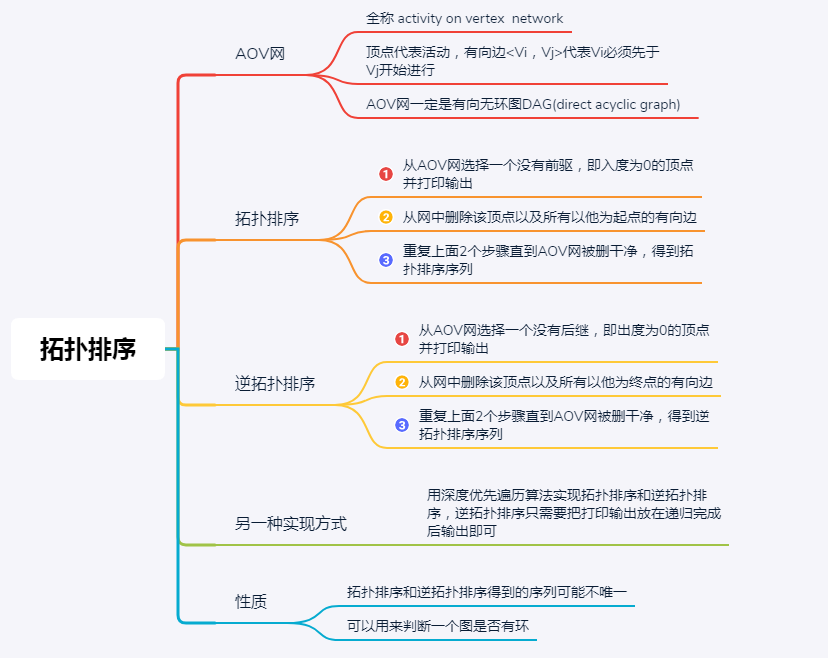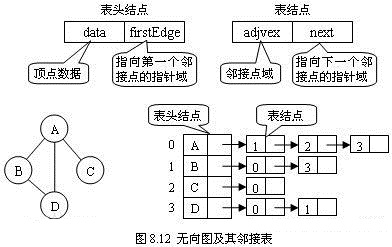• 拓扑排序算法，用C++写的，有注释，适合初学者。拓扑排序
• 主要为大家详细介绍了C++实现拓扑排序，文中示例代码介绍的非常详细，具有一定的参考价值，感兴趣的小伙伴们可以参考一下C++ 拓扑排序
• 拓扑排序 //伪代码 typedef struct ArcNode{ int adjvex;//该边指向的顶点的下标 struct ArcNode *nextarc;//下条边指针 }ArcNode; typedef struct VNode{ char data;//顶点信息 例如a,b,c... ArcNode *...

拓扑排序//伪代码
typedef struct ArcNode{
struct ArcNode *nextarc;//下条边指针
}ArcNode;
typedef struct VNode{
char data;//顶点信息 例如a,b,c...
ArcNode *firstarc;
typedef struct{
int vexnum;
int arcnum;
}Graph;//一般图都要有统计边数和顶点数，所以再写一层结构体
//
Graph graph;// 邻接表实现
bool TopologicalSort(Graph graph){
Stack stack;
InitStack(stack);
int indegree[];//初始保存每个顶点的入度数

int print[];//保存顶点输出序列

// 循环遍历indegree数组，将入度为0进栈，或者队列，随便其他都可以，方便读取和删除就可以
for(int i = 0; i<graph.vexnum; i++){
if(degree[i]==0)
Push(stack, i);
}

int v = 0;
int i = 0, j = 0;
// 入度为0的进栈，出栈访问，然后把其他入度为0的入栈，直到栈为空，最后判断print数组的顶点个数，和实际图的顶点个数是否相等
while(!IsEmpty(stack)){
Pop(S, i);
print[j++] = i;
// p 边结点指针
// 删掉该顶点，意味着要把indegree数组对应-1
for(p = graph.vertices[i].firstarc; p!=NULL;p->nextarc){
indegree[v]--;
// 判断是否入度为0 进栈，注意只有与上面被删结点指向的顶点入度才有可能为0，不需要检查其他的顶点
if(indegree[v]==0)
Pop(S, v);
}
}

if(j==graph.vexnum)
return true;
else
return false;
}

展开全文c++ 数据结构 算法
• 拓扑排序C++实现

万次阅读 多人点赞 2016-09-21 16:00:30
对一个有向无环图(Directed Acyclic Graph, DAG)G进行拓扑排序，是将G中所有顶点排成一个线性序列，使得图中任意一对顶点u和v，若 ∈E(G)，则u在线性序列中出现在v之前。 通常，这样的线性序列称为满足拓扑次序...

一.定义

对一个有向无环图(Directed Acyclic Graph, DAG)G进行拓扑排序，是将G中所有顶点排成一个线性序列，使得图中任意一对顶点u和v，若<u，v> ∈E(G)，则u在线性序列中出现在v之前。

通常，这样的线性序列称为满足拓扑次序(Topological Order)的序列，简称拓扑序列

注意:

1)只有有向无环图才存在拓扑序列;

2)对于一个DAG,可能存在多个拓扑序列;

如:该DAG的拓扑序列为 A B C D 或者 A C B D而此有向图是不存在拓扑序列的，因为图中存在环路

二.拓扑序列算法思想

(1)从有向图中选取一个没有前驱(即入度为0)的顶点，并输出之;

(2)从有向图中删去此顶点以及所有以它为尾的弧;

重复上述两步，直至图空，或者图不空但找不到无前驱的顶点为止。

三.代码实现

采用邻接表实现，邻接表表示请见下图。#include <iostream>
#include <fstream>
#include <stack>
#include <cstring>
using namespace std;

#define MAX_VERTEX_NUM 26

typedef struct ArcNode{
struct ArcNode *nextarc;
ArcNode(){nextarc=NULL;}
}ArcNode;

typedef struct VNode{
int data;
ArcNode *firstarc;
VNode(){firstarc=NULL;}

typedef struct{
int vexnum,arcnum;
}ALGraph;

bool TopologicalSort(ALGraph G,int *indegree)
{
stack<int> s;

int i,k;
for(i=1;i<G.vexnum+1;i++)
{
if(!indegree[i])
s.push(i);
}

int count=0;
ArcNode *p;
while(!s.empty())
{
i = s.top();
s.pop();
cout<<G.vertices[i].data<<"->";
count++;
for(p=G.vertices[i].firstarc;p;p=p->nextarc)
{
indegree[k]--;
if(!indegree[k])
s.push(k);
}
}

if(count<G.vexnum)
return false;

return true;
}

int main()
{
int i;
ALGraph g;
cout<<"载入图中..."<<endl;
ifstream fin("in.txt");
fin>>g.vexnum>>g.arcnum;
for(i=1;i<g.vexnum+1;i++)
g.vertices[i].data = i;

int b,e;
ArcNode *p;
int *indegree = new int[g.vexnum+1];
//注意 int *a=new int(n);  申请一个整型变量空间，赋初值为n，并定义一个整型指针a指向该地址空间
//int *indegree=(int *)malloc(sizeof(int)*(g.vexnum+1));
memset(indegree,0,sizeof(int)*(g.vexnum+1));
for(i=1;i<g.arcnum+1;i++)
{
fin>>b>>e;
cout<<"第"<<i<<"条边："<<b<<"->"<<e<<endl;

p = new ArcNode();
p->nextarc = g.vertices[b].firstarc;
g.vertices[b].firstarc = p;
indegree[e]++;
}

if(TopologicalSort(g,indegree))
cout<<"正常完成！"<<endl;
else
cout<<"该有向图有回路！"<<endl;

return 0;
}

两组测试数据：

1）有环

4 4
1 2
2 3
3 4
4 2

2）无环

12 16
1 2
1 3
2 3
1 4
3 5
4 5
11 6
5 7
3 7
3 8
6 8
9 10
9 11
9 12
10 12
1 12

展开全文C++ 拓扑排序
• 拓扑排序与关键路径，在日常生活中，一项大的工程可以看作是由若干个子工程（这些子工程称为“活动” ）组成的集合，这些子工程（活动）之间必定存在一些先后关系，即某些子工程（活动）必须在其它一些子工程（活动...拓扑排序
• 拓扑排序C++实现 思路 拓扑排序在作者看来本质上是每次寻找入度为0节点的图的遍历，所以在进行拓扑排序的时候我们首先要建立一个图，由于是要进行拓扑排序，所以我们要建立的图一定是一个有向图。然后每次去查找邻接...

拓扑排序C++实现

思路

拓扑排序在作者看来本质上是每次寻找入度为0节点的图的遍历，所以在进行拓扑排序的时候我们首先要建立一个图，由于是要进行拓扑排序，所以我们要建立的图一定是一个有向图。然后每次去查找邻接矩阵中入度为0的元素输出，然后把这个元素映射过去的arr矩阵中相应的行元素全部置为0，相当于切断此元素指向其他元素的边。然后不断重复此操作即可。
下面给出实现的代码。

#include<iostream>
#include<queue>
#define MAX 100
int arr[MAX][MAX];
int flag[MAX];//记录是否已经访问过该元素
using namespace std;
int findloc(char a[], char c,int n)//查找字符c在数组中的位置
{
int i;
for (i = 0; i < n; i++)
{
if (a[i] == c)
break;
}
return i;
}
int findinnum(char a[], char c, int n)//统计入度数
{
int loc = findloc(a, c, n);
int cnt = 0;
for (int i = 0; i < n; i++)
{
if (arr[i][loc] == 1)
cnt++;
}
return cnt;
}
void creategraph(char a[], int n,int arc)//创建一个数据结构为邻接矩阵的图
{
cout << "输入每条边相邻的两个节点：(前为起点)" << endl;
char c1, c2;
int loc1, loc2;
for(int i=0;i<n;i++)
for (int j = 0; j < n; j++)
{
arr[i][j] = 0;
}
for (int i = 0; i < arc; i++)
{
cin >> c1 >> c2;
loc1 = findloc(a, c1, n);
loc2 = findloc(a, c2, n);
arr[loc1][loc2] = 1;
}
cout << "当前邻接矩阵为：" << endl;
for (int i = 0; i < n; i++)
{
for (int j = 0; j < n; j++)
{
cout << arr[i][j] << " ";
}
cout << endl;
}
}
void topsort(char a[], int n)//拓扑排序
{
cout << endl << "拓扑排序：";
for (int i = 0; i < n; i++)
{
for (int j = 0; j < n; j++)
{
if (findinnum(a, a[j], n) == 0 && flag[j]==1)
{
cout << a[j] << " ";
flag[j] = 0;
for (int k = 0; k < n; k++)
{
arr[j][k] = 0;
}
break;
}
}
}
}
int main()
{
int n, arc;
cout << "输入顶点数，边数:";
cin >> n >> arc;
char* a = new char[n];
cout << "输入各个顶点名称：";
for (int i = 0; i < n; i++)
{
flag[i] = 1;
}
for (int i = 0; i < n; i++)
{
cin >> a[i];
}
for (int i = 0; i < n; i++)
for (int j = 0; j < n; j++)
{
arr[i][j] = 1;
}
creategraph(a, n, arc);
topsort(a, n);
return 0;
}

展开全文c++ 数据结构 算法 排序算法
• 拓扑排序不唯一 算法 依次拿掉入度（指向自己的箭头个数）为零的节点（起点），并把起点的邻接点的入度减一。 重复以上操作，直到不存在入度为零的点（起点）。 如果最后仍然剩余节点，说明图中必然有环。 准备...

理论准备

• 优先级的调度问题，做饭先烧水，切菜先买菜，炒菜先放油，活动之间有先后限制关系。
• 把必须先准备的事放到前面，需要大量铺垫的活动放到后面，得到一个序列就是拓扑排列。
• 拓扑排序不唯一算法

• 依次拿掉入度（指向自己的箭头个数）为零的节点（起点），并把起点的邻接点的入度减一。
• 重复以上操作，直到不存在入度为零的点（起点）。
• 如果最后仍然剩余节点，说明图中必然有环。

准备：图的度数类API

• Degrees（Digraph G） 构造函数
• int indegree（int v）v的入度
• int outdegree（int v）v的出度
• list<int> sources()所有起点的集合
• list<int> sinks()所有终点的集合
• bool isMap()G是一幅映射吗？

定义：入度为零的点是起点，出度为零的点为终点，一个图允许出现自环且每个顶点的出度为1的有向图叫做映射（从0到V-1之间的整数到他们自身的函数）

实现degrees

得到 Digraph.h 点这里

#pragma once
#include<queue>
#include"Digraph.h"
class Degrees
{
public:
Degrees(Digraph& G);

int indegree(int v) { return m_indegree->at(v); }
int outdegree(int v) {
return m_outdegree->at(v);
}

list<int>* sources();
list<int>* sinks();

bool isMap();
private:
vector<int>* m_indegree=nullptr;
vector<int>* m_outdegree=nullptr;
};

list<int>* TopSortByQueue(Digraph& G);
void testForTopSortByQ();

队列拓扑排序 Degrees.cpp 见注释

#include "Degrees.h"

Degrees::Degrees(Digraph& G)
{
int n = G.V();
m_indegree = new vector<int>(n, 0);
m_outdegree = new vector<int>(n, 0);

for (int i = 0; i < n; ++i) {
++m_indegree->at(*it);
}
}
}

list<int>* Degrees::sources()
{
list<int>* ans = new list<int>();
for (int i = 0; i < m_indegree->size();++i) {
if (m_indegree->at(i) == 0) {
ans->push_back(i);
}
}
return ans;
}

list<int>* Degrees::sinks()
{
list<int>* ans = new list<int>();
for (int i = 0; i < m_outdegree->size(); ++i) {
if (m_outdegree->at(i) == 0) {
ans->push_back(i);
}
}
return ans;
}

bool Degrees::isMap()
{
bool is_map = true;
for (int i = 0; i < m_outdegree->size(); ++i) {
if (m_outdegree->at(i) != 1) {
is_map = false;
break;
}
}
return is_map;
}

list<int>* TopSortByQueue(Digraph& G)
{
list<int>* topSortList = new list<int>();

vector<bool>* marked = new vector<bool>(G.V(), false);
vector<int>* indegrees = new vector<int>(G.V(), 0);
queue<int>* inQ = new queue<int>();

//取出入度放入数组indegrees
Degrees dg(G);
for (int i = 0; i < G.V(); ++i) {
indegrees->at(i) = dg.indegree(i);
}

//将起点（入度为零的点）入队
list<int>* sources = dg.sources();
forIt(sources) {
inQ->push(*it);
}

//队列不空
while (!inQ->empty())
{
//取出 标记 写到topSortList
int now = inQ->front(); inQ->pop();
marked->at(now) = true;
topSortList->push_back(now);
//修改邻接点的入度，把新起点入队
int nxt = *it;
if (!marked->at(nxt)) {
indegrees->at(nxt)--;
if (indegrees->at(nxt) == 0) {
inQ->push(nxt);
}
}

}
}

}

void testForTopSortByQ()
{
Digraph G("tinyDAG.txt");
list<int>* topSlist = TopSortByQueue(G);
forIt(topSlist) {
out(*it);
}
hh;
}
/*
2 8 0 3 7 1 5 6 9 4 11 10 12
*/

测试图依次取出起点，得到拓扑序列：

2 8 0 3 7 1 5 6 9 4 11 10 12拓扑排序的其他思路 点这里

展开全文c++ 队列 数据结构 拓扑排序
• 拓扑排序C++

2022-01-02 21:55:08
拓扑排序 对一个有向无环图(Directed Acyclic Graph简称DAG)G进行拓扑排序，是将G中所有顶点排成一个线性序列，使得图中任意一对顶点u和v，若边<u,v>∈E(G)，则u在线性序列中出现在v之前。通常，这样的线性...算法
• 利用队列广度优先（BFS）实现拓扑排序 class Solution { public: vector<int> findOrder(int numCourses, vector<vector<int>>& prerequisites) { //构建邻接表，存储有向图 vector<...
• 如果队列为空时入过队的结点数目恰好为N，说明拓扑排序成功，图G为有向无环图;否则，拓扑排序失败，图G中有环。 可使用邻接表实现拓扑排序。显然，由于需要记录结点的入度，因此需要额外建立一个数组inDegree[MAXV]...算法 c++ 数据结构 排序算法
• 文章目录数据结构C++——拓扑排序一、前言二、拓扑排序的概念及作用三、拓扑排序的实现①拓扑排序的实现原理②拓扑排序中FindInDegree()函数的实现③拓扑排序的代码实现④完整测试代码四、总结 一、前言 拓扑排序...数据结构 c++ 算法
• 拓扑排序c++

2021-07-31 17:00:03
拓扑排序判断无向图中是否有环 #include<iostream> #include<vector> #include<queue> using namespace std; void TopSort(vector<vector<int>>& grid, vector<int>&...
• 拓扑排序算法C++实现

千次阅读 2018-01-04 19:18:44
拓扑排序算法，基本思想: 1、从有向图中选取一个没有前驱（入度为0）的顶点，并输出之 2、从有向图中删去此顶点以及所有以它为尾的弧 3、重复上述两步，直至图空，或者图不空但找不到无前驱的顶点为止 数据...算法 拓扑排序
• 既然是一种排序，那么肯定是按照某种规则进行排序，那么这么想的话，先了解基本知识，再来实战演练 1. AOV网（Activity On Vertex Network)【顶点——表示活动】 是一个——有向无回路的图 顶点——表示活动 用弧...排序算法 有向图 数据结构 拓扑排序
• C++拓扑排序

2021-02-18 20:48:14
对于一个有向无环图（DAG），求拓扑排序最常见的方法: ① 从DAG图中选择入度为0的顶点，并输出。 ② 从图中删除该入度为0的顶点及所有以它为起点的边。 ③ 重复（1）和（2）直到当前图为空，或者图不存在入度为0...
• 对于有向图进行拓扑排序，图使用邻接矩阵的存储结构。图 拓扑排序
• 图的拓扑排序（C++实现）

千次阅读 2019-03-08 10:37:03
/// 我们把此序列叫做拓扑序列(Topological order)，由AOV网构造拓扑序列的过程叫做拓扑排序(Topological sort)。 /// AOV网的拓扑序列不是唯一的，满足上述定义的任一线性序列都称作它的拓扑序列。 /// 二者的...
• AOV-网： 一个无环的有向图称作有向无环图(Directed ...检测的办法是对有向图的顶点进行拓扑排序，若网中所有顶点都在它的拓扑有序序列中，则该AOV-网中必定不存在环，否则有环。 除了用拓扑排序判断图有无环外，数据结构 算法
• 有向无环图才有拓扑排序。问题，如果判断一个有向图是否有环？点这里 拓扑排序的背后是约束关系，优先调度的问题首先被考虑。简单的，你应该先学习十进制数字再研究数论。问题，如何得到拓扑排序呢？点这里 拓扑...c++ 拓扑排序
• C++实现图的拓扑排序

千次阅读 2020-05-17 14:55:08
另一个正确的排序是 [0,2,1,3] 。 深度优先遍历dfs class Solution { private: // 存储有向图 vector<vector<int>> edges; // 标记节点的状态。0 = 未搜索，1 = 搜索中，2 = 已完成 vector<int> visited; // 数组...
• 代码如下: #include<iostream> #include <vector> #include <string> #include <stack> using namespace std; const int N = 10010; int in[N]; vector<int>... n .c++ 算法 数据结构 拓扑排序
•   有向图的拓扑排序，是这么个意思：对图的顶点进行排序，从左到右输出。对于图里任意一条边，边的起点都在终点的左面。对于排序中的任意两个顶点，这俩顶点要么没有边相连，要么左边的顶点一定是起点，右边的点是...数据结构 算法 c语言 c++ 图搜索算法
•  拓扑排序是将有向无环图G的所有顶点排成一个线性序列，使得对图G中的任意两个顶点u、v，如果存在边u-&gt;v，那么在序列中u一定在v前面，这个序列又被称为拓扑序列。 如下图：结点0和1没有前驱节点，可以任意...
• 请输出任意一个该有向图的拓扑序列，如果拓扑序列不存在，则输出−1。 若一个由图中所有点构成的序列AA满足：对于图中的每条边(x,y)，x在A中都出现在y之前，则称A是该图的一个拓扑序列。 输入格式 第一行包含两个...c++ 算法 数据结构
• C++语言实现-拓扑排序

千次阅读 2018-11-14 20:18:00
1、拓扑排序的概念 对一个有向无环图(Directed Acyclic Graph简称DAG)G进行拓扑排序，是将G中所有顶点排成一个线性序列，使得图中任意一对顶点u和v，若边(u,v)∈E(G)，则u在线性序列中出现在v之前。 2、拓扑排序...
• 图的拓扑排序（有向图），用一个矩阵存储，环境为VC6.0...

拓扑排序c++c++ 订阅